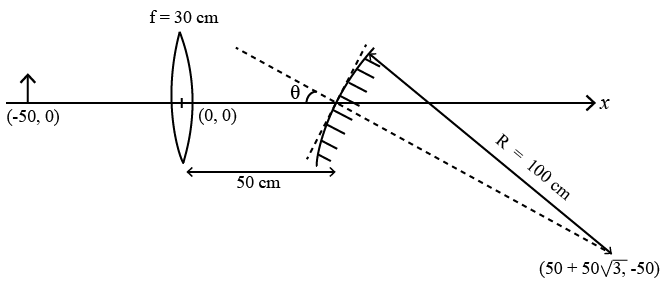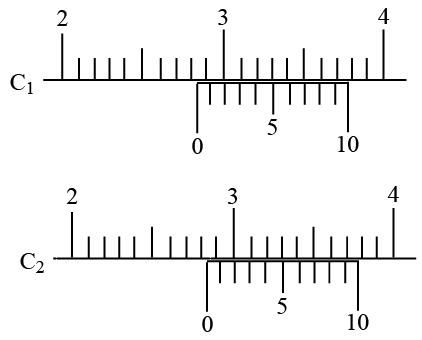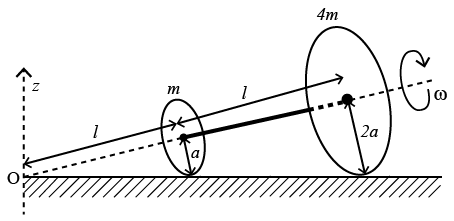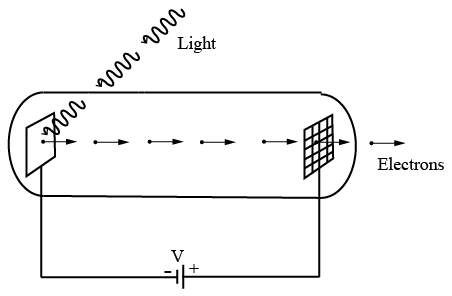Instructions

For the following questions answer them individually

Question 1

# The electrostatic energy of Z protons uniformly distributed throughout a spherical nucleus of radius R is given by$$E = \frac{3}{5}\frac{Z(Z - 1)e^2}{4 \pi ε_0 R}$$The measured masses of the neutron, $$^1_1H, ^{15}_7N$$ and $$^{15}_8O$$ are 1.008665 u, 1.007825 u, 15.000109 u and 15.003065 u,respectively. Given that the radii of both the $$^{15}_7N$$ and $$^{15}_8O$$ nuclei are same, $$1 u = 931.5 MeV/c^2$$ (c is the speed oflight) and $$\frac{e^2}{(4 \pi ε_0)} = 1.44 MeV fm$$. Assumingthat the difference between the binding energies of $$^{15}_7 N$$ and $$^{15}_8 O$$ is purely due to the electrostatic energy, the radius of either of the nuclei is$$(1 fm = 10^{-15}m)$$Question 2

# An accident in a nuclear laboratory resulted in deposition of a certain amount of radioactive material of half-life 18 days inside the laboratory. Tests revealed that the radiation was 64 times more than the permissible level required for safe operation of the laboratory. What is the minimum number of days after which the laboratory can be considered safe for use?Question 3

# A gas is enclosed in a cylinder with a movable frictionless piston. Its initial thermodynamic state at pressure $$P_i = 10^5 Pa$$ and volume $$V_i = 10^{-3} m^3$$ changes to a final state at $$P_f = \left(\frac{1}{32}\right) \times 10^5 Pa$$ and $$V_f = 8 \times 10^{-3} m^3$$ in an adiabatic quasi-static process, such that $$P^3V^5$$ = constant. Consider another thermodynamic process that brings the system from the same initial state to the same final state in two steps: an isobaric expansion at $$P_i$$. followed by an isochoric (isovolumetric) process at volume $$V_f$$. The amount of heat supplied to the system in the two-step process is approximatelyQuestion 4

# The ends Q and of two thin wires, PQ and RS, are soldered (joined) together. Initially each of the wires has a length of 1 m at $$10^\circ C$$. Now the end P is maintained at $$10^\circ C$$, while the end S is heated and maintained at $$400^\circ C$$. The system is thermally insulated from its surroundings. If the thermal conductivity of wire PQ is twice that of the wire RS and the coefficient of linear thermal expansion of PQ is $$1.2 \times 10^{-5}K^{-1}$$ the change in length of the wire PQ isQuestion 5

# A small object is placed 50 cm to the left of a thin convex lens of focal length 30 cm. A convex spherical mirror of radius of curvature 100 cm is placed to the right of the lens at a distance of 50 cm. The mirror is tilted such that the axis of the mirror is at an angle $$\theta = 30^\circ$$ to the axis of the lens, as shown in the figure.If the origin of the coordinate system is taken to be at the center of the lens, the coordinates (in cm) of the point (x,y) at which the image is formed areQuestion 6

# There are two Vernier calipers both of which have 1 cm divided into 10 equal divisions on the main scale. The Vernier scale of one of the calipers $$(C_1)$$ has 10 equal divisions that correspond to 9 main scale divisions. The Vernier scale of the other caliper $$(C_2)$$ has 10 equal divisions that correspond to 11 main scale divisions. The readings of the two calipers are shown in the figure. The measured values (in cm) by calipers $$C_1$$ and $$C_2$$, respectively, areQuestion 7

# Two thin circular discs of mass m and 4m, having radii of a and 2a, respectively, are rigidly fixed by a mass less, rigid rod of length $$l = 24\sqrt{24} a$$ through their centers. This assembly is laid on a firm and flat surface, and set rolling without slipping on the surface so that the angular speed about the axis of the rod is $$\omega$$. The angular momentum of the entire assembly about the point ‘O’ is $$\overrightarrow{L}$$ (see the figure). Which of the following statement(s) is(are) true?Question 8

# Light of wave length $$\lambda_{ph}$$ falls on a cathode plate inside a vacuumtube as shown in the figure. The work function of the cathode surface is $$\phi$$ and the anode is a wire mesh of conducting material kept at a distance d from the cathode. A potential difference V is maintained between the electrodes. If the minimum de Broglie wavelength of the electrons passing through the anode is $$\lambda_{e}$$, which of the following statement(s) is(are) true?Question 9

# In an experiment to determine the acceleration due to gravity g, the formula used for the time period of a periodic motion is $$T = 2 \pi \sqrt{\frac{7(R - r)}{5g}}$$. The values of R and r are measured to be $$(60 \pm 1)$$ mm and $$(10 \pm 1)$$ mm, respectively. In five successive measurements, the time period is found to be 0.52 s, 0.56 s, 0.57 s, 0.54 s and 0.59 s. The least count of the watch used for the measurement of time period is 0.01 s. Which of the following statement(s) is(are) true?Question 10

# Consider two identical galvanometers and two identical resistors with resistance R. If the internal resistance of the galvanometers $$R_c < \frac{R}{2}$$, which of the following statement(s) about any one of the galvanometers is(are) true?OR